Problem title
Difficulty
Avg time to solve

Kruskal’s Minimum Spanning Tree Algorithm
Moderate
--
Mike and Mobile
Moderate
35 mins
Star Pattern
Easy
--
Easy
15 mins
Find K-th smallest Element in BST
Easy
15 mins
Minimum Insertions
Moderate
20 mins
Largest Submatrix with Equal Number of 0's and 1's
Easy
15 mins
Binary Tree Zigzag Traversal
Easy
15 mins
Minimum Time To Solve The Problems
Hard
50 mins
Sort a half-sorted array
Easy
10 mins5

# Binary Tree Zigzag Traversal

Difficulty: EASY
Avg. time to solve
15 min
Success Rate
85%

Problem Statement

#### You have been given a Binary Tree of 'N' nodes, where the nodes have integer values. Your task is to print the zigzag traversal of the given tree.

##### Note:
``````In zigzag order, level 1 is printed from left to right fashion, level 2 is printed from right to left. and level 3 is printed from left to right again, and so on…..
``````
##### For example:
``````For the given binary tree
``````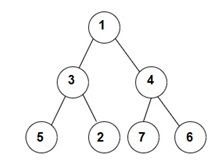``````The zigzag  traversal is [1, 4, 3, 5, 2, 7, 6]
``````
##### Input Format:
``````The first line contains an integer 'T' which denotes the number of test cases or queries to be run. Then the 'T' test cases follow.

The first line of each test case contains elements of the tree in the level order form. The line consists of values of nodes separated by a single space. In case a node is null, we take -1 in its place.

For example, the input for the tree depicted in the below image
``````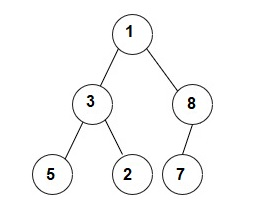``````1
3 8
5 2 7 -1
-1 -1 -1 -1 -1 -1
``````

#### Explanation :

``````Level 1 :
The root node of the tree is 1

Level 2 :
Left child of 1 = 3
Right child of 1 = 8

Level 3 :
Left child of 3 = 5
Right child of 3 = 2
Left child of 8 =7
Right child of 8 =  null (-1)

Level 4 :
Left child of 5 = null (-1)
Right child of 5 = null (-1)
Left child of 2 = null (-1)
Right child of 2 = null (-1)
Left child of 7 = null (-1)
Right child of 7 = null (-1)

The first not-null node(of the previous level) is treated as the parent of the first two nodes of the current level. The second not-null node (of the previous level) is treated as the parent node for the next two nodes of the current level and so on.
The input ends when all nodes at the last level are null(-1).
``````
##### Note :
``````The above format was just to provide clarity on how the input is formed for a given tree.
The sequence will be put together in a single line separated by a single space. Hence, for the above-depicted tree, the input will be given as:
1 3 8 5 2 7 -1 -1 -1 -1 -1 -1 -1
``````
##### Output Format:
``````For each test case, print a single line containing all the nodes value in zigzag order traversal separated by a single space in a separate line.
``````
##### Note :
``````You do not need to print anything, it has already been taken care of. Just implement the given function.
``````
##### Constraints:
``````1 <= 'T' <= 100
0 <= 'N' <= 10^3
0 <= 'NODES' <= 10^9

Where NODES represent any node value

Time Limit: 1sec
``````
##### Sample Input 1:
``````2
1 2 3 -1 -1 -1  6 -1 -1
1 2 3 -1 -1 -1 -1
``````
##### Sample Output 1:
``````1 3 2 6
1 3 2
``````
##### Explanation of Sample Input 1:
`````` For the first test case, the given binary tree is shown below.
``````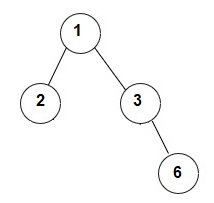``````zigzag traversal of given tree = [1, 3, 2, 6]

For the second test case, the given binary tree is shown below.
``````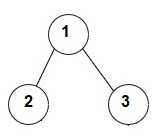``````ZigZag traversal of given tree = [1, 3, 2]
``````
##### Sample Input 2:
``````1
1 2 4 5 3 -1 -1 -1 -1 -1 -1
``````
##### Sample Output 2:
``````1 4 2 5 3
``````
##### Explanation of Sample Input 2:
``````For the first test case, the given binary tree is shown below.
``````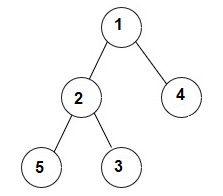``````ZigZag traversal of given tree = [1, 4, 2, 5, 3]
``````Console